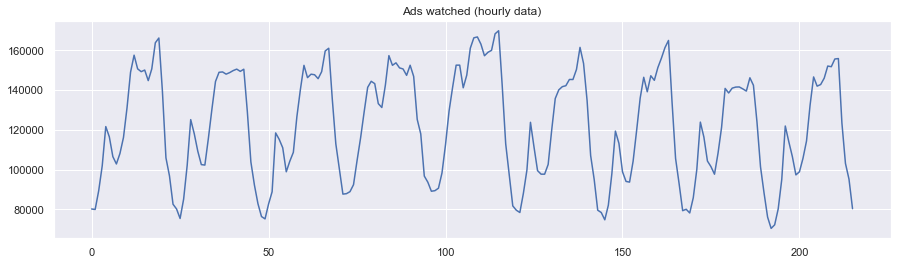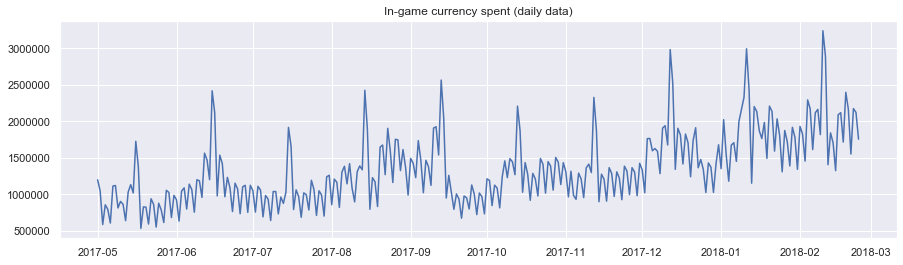## 时间序列处理与应用¶

• 时间序列
• SARIMA
• 线性回归
• XGBoost
• Prophet

### 时间序列数据介绍¶

In :
import warnings
from itertools import product

import matplotlib.pyplot as plt
import numpy as np
import pandas as pd
import scipy.stats as scs
import seaborn as sns
import statsmodels.api as sm
import statsmodels.formula.api as smf  # 统计与计量
import statsmodels.tsa.api as smt
from dateutil.relativedelta import relativedelta
from scipy import stats
from scipy.optimize import minimize  # 优化函数
from tqdm import tqdm_notebook

sns.set()
%matplotlib inline
warnings.filterwarnings("ignore")


In :
ads = pd.read_csv("../data/ads.csv", index_col=["Time"], parse_dates=["Time"])

Out:
((216, 1), (300, 1))

In :
plt.figure(figsize=(15, 4))
plt.grid(True)
plt.show()In :
plt.figure(figsize=(15, 4))
plt.plot(currency.GEMS_GEMS_SPENT)
plt.title("In-game currency spent (daily data)")
plt.grid(True)
plt.show()